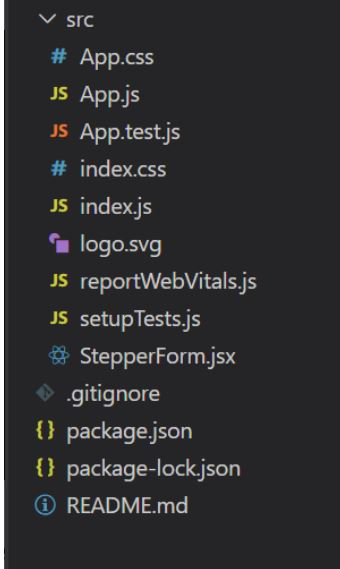Open In App

# How to render an array of objects in ReactJS ?

ReactJS is a popular JavaScript library for building user interfaces, and it provides a powerful way to render dynamic content. When working with arrays of objects in React, you may often need to display them in your UI. In this article, we will explore how to render an array of objects in ReactJS and provide some best practices along the way. In this article, we will learn about “How one can render an array of objects in React?”

Array of objects: It stores multiple values in a single variable. The object can contain anything in the real world, such as person names, cars, and game characters. Objects are very easy to use in some situations if you know where the data is being processed. The character set of objects is known as Properties. Properties of an object can be called by using DOT(“.”) notation and (“[]”) notation.
Syntax:

```let object=[
{
"property1":"value1",
"property2":"value2",
}
]```

Now, let’s create a React application to learn How to render an array of objects.

Creating React Application:

Step 1: Create a React application using the following command.

`npx create-react-app foldername`

Step 2: Once it is done, change your directory to the newly created application using the following command.

`cd foldername`

Project Structure: The project should look like this:How we will render an Array of Objects?

We will use the map function to access each object from the array.
The map() method in JavaScript creates an array by calling a specific function on each element present in the parent array. It is a non-mutating method. Generally, the map() method is used to iterate over an array and call functions on every element of the array.
Syntax:

`array.map(function(currentValue, index, arr), thisValue)`

Parameters: This method accepts two parameters, as mentioned above and described below:

• function(currentValue, index, arr): It is a required parameter, and it runs on each element of the array. It contains three parameters, which are listed below:
• currentValue: It is a required parameter, and it holds the value of the current element.
• index: It is an optional parameter, and it holds the index of the current element.
• arr: It is an optional parameter, and it holds the array.
• thisValue: It is an optional parameter and is used to hold the value passed to the function.

Return Value: It returns a new array and elements of arrays are the result of the callback function.

Example 1: In this example, we will simply create an array of objects that contains states and their capitals. We will render them on an unordered list. States are in red and bold.

## Javascript

 `import React from ``'react'``function` `RenderingArrayOfObjects() {``    ``const data = [``        ``{``            ``"State"``: ``"Uttar Pradesh"``,``            ``"Capital"``: ``"Lucknow"``        ``},``        ``{``            ``"State"``: ``"Gujarat"``,``            ``"Capital"``: ``"Gandhinagar"``        ``},``        ``{``            ``"State"``: ``"Karnataka"``,``            ``"Capital"``: ``"Bengaluru"``        ``},``        ``{``            ``"State"``: ``"Punjab"``,``            ``"Capital"``: ``"Chandigarh"``        ``},``        ``{``            ``"State"``: ``"Maharashtra"``,``            ``"Capital"``: ``"Mumbai"``        ``}``    ``]``    ``const listItems = data.map(``        ``(element) => {``            ``return` `(``                ``
``                    ``
• ``                        ``{element.State}``                    ``
• ``                    ``
• {element.Capital}
• ``                ``
``            ``)``        ``}``    ``)``    ``return` `(``        ``
``            ``{listItems}``        ``
``    ``)``}``function` `App() {``    ``return` `(``        ``
``            ``
``                ``

``                    ``GeeksforGeeks``                ``

``                ``

Rendering Array of Objects

``                ``

``                ````            ``
``        ``
``    ``);``}` `export ``default` `App;`

Output:Example 2: In this example, we will map an Array of objects containing student Marks into a Table.

Note: To run the below example, you need to install react-bootstrap and bootstrap.

`npm install react-bootstrap bootstrap@5.1.3 bootstrap`

## Javascript

 `import React from ``'react'``;``import Table from ``'react-bootstrap/Table'``;``import ``'bootstrap/dist/css/bootstrap.min.css'``;` `function` `RenderingArrayOfObjects() {``    ``const data = [``        ``{``            ``"Name"``: ``"Nikita"``,``            ``"Marks"``: ``"98"``,``            ``"Phone"``: ``"123"``        ``},``        ``{``            ``"Name"``: ``"Pratiksha"``,``            ``"Marks"``: ``"96"``,``            ``"Phone"``: ``"127"``        ``},``        ``{``            ``"Name"``: ``"Muskan"``,``            ``"Marks"``: ``"97"``,``            ``"Phone"``: ``"163"``        ``},``        ``{``            ``"Name"``: ``"Nishi"``,``            ``"Marks"``: ``"95"``,``            ``"Phone"``: ``"193"``        ``},``        ``{``            ``"Name"``: ``"Himanshu"``,``            ``"Marks"``: ``"78"``,``            ``"Phone"``: ``"120"``        ``}``    ``]``    ``const tableRows = data.map(``        ``(element) => {``            ``return` `(` `                ``
{element.Name}{element.Marks}{element.Phone}
``                    ````                    ````                    ````                ``` `            ``)``        ``}``    ``)``    ``return` `(``        ``
` `            ````                ``
NameMarksPhone
``                    ````                        ````                        ````                        ````                    ````                ````                ````                    ``{tableRows}``                ````            ``` `        ````    ``)``}``function` `App() {``    ``return` `(``        ``
``            ``
``                ``

GeeksforGeeks

``                ``

Rendering Array of Objects

` `                ``

``                ``` `            ``
``        ``
``    ``);``}` `export ``default` `App;`

Output: